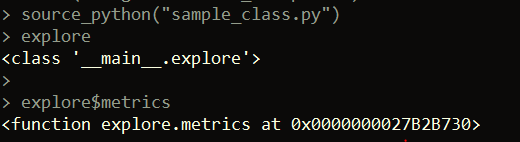Want to share your content on R-bloggers? click here if you have a blog, or here if you don't.## Background

This post is going to talk about how to import Python classes into R, which can be done using a really awesome package in R called reticulate. reticulate allows you to call Python code from R, including sourcing Python scripts, using Python packages, and porting functions and classes.

To install reticulate, we can run:

```install.packages("reticulate")

```

## Creating a Python class

Let’s create a simple class in Python.

```import pandas as pd

# define a python class

class explore:

def __init__(self, df):

self.df = df

def metrics(self):

desc = self.df.describe()

return desc

def dummify_vars(self):

for field in self.df.columns:

if isinstance(self.df[field], str):

temp = pd.get_dummies(self.df[field])

self.df = pd.concat([self.df, temp], axis = 1)

self.df.drop(columns = [field], inplace = True)

```

## Porting the Python class to R

There’s a couple ways we can can port our Python class to R. One way is by sourcing a Python script defining the class. Let’s suppose our class is defined in a script called “sample_class.py”. We can use the reticulate function source_python.

```# load reticulate package
library(reticulate)

# inside R, source Python script
source_python("sample_class.py")

```

Running the command above will not only make the class we defined will available to us in our R session, but would also make any other variables or functions we defined in the script available as well (if those exist). Thus, if we define 10 classes in the script, all 10 classes will be available in the R session. We can refer to any specific method defined in a class using R’s “\$” notation, rather than the “dot” notation of Python.```result <- explore(iris)

# get summary stats of data
result\$metrics()

# create dummy variables from factor / character fields
# (just one in this case)
result\$dummify_vars()

```

One other note is that when you import a class from Python, the class becomes a closure in R. You can see this by running R’s typeof function:

```typeof(explore) # closure

```

## Using R markdown to switch between R and Python

Another way of using a Python class in R is by using R Markdown. This feature is available in RStudio v. 1.2+ and it allows us to write chunks of R code followed by chunks of Python code, and vice-versa. It also lets us pass variables, or even classes from Python to R. Below, we write the same code as above, but this time using chunks in an R Markdown file. When we write switch between R and Python chunks in R Markdown, we can reference Python objects (including classes) by typing py\$name_of_object, where you just need to replace name_of_object with whatever you’re trying to reference from the Python code. In the below case, we reference the explore class we created by typing py\$explore.

```
```{r}

library(reticulate)

```

```{python}

import pandas as pd

# define a python class

class explore:

def __init__(self, df):

self.df = df

def metrics(self):

desc = self.df.describe()

return desc

def dummify_vars(self):

for field in self.df.columns:

if isinstance(self.df[field], str):

temp = pd.get_dummies(self.df[field])

self.df = pd.concat([self.df, temp], axis = 1)

self.df.drop(columns = [field], inplace = True)

```

```{r}

py\$explore

```

```

## Example with class and instance variables

Now, let’s look at another example. Below, we create a Python class in a file called “sample_class2.py” that has an instance variable (value) and a class variable (num).

```class test:

def __init__(self, value):
self.value = value

def class_var(self):
test.num = 10

source_python("sample_class2.py")

check = test(5)

check\$value

check\$num # error because class_var hasn't been called yet

check\$class_var()

check\$num # works now

test\$num

```